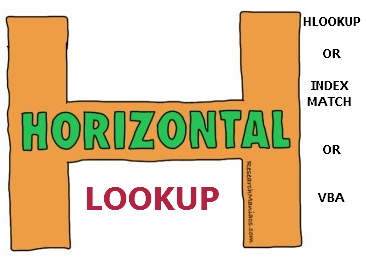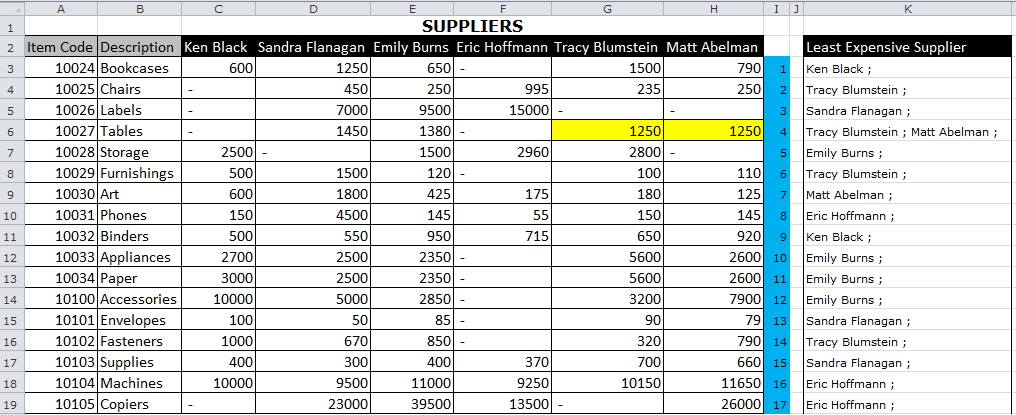# HLOOKUP, INDEX & MATCH OR VBA

Having done a series on Verticle lookup, it is only fair to touch on the Horizontal lookup.

HLOOKUP function is the most popular function for this kind of lookup but it has a number of limitations and thus one may need to know a few other ways you can carry out a Horizontal lookup.

For example, given below data of suppliers, items supplied and prices for each supplier, Can you extract the least expensive supplier(s)?## VBA

We are going to use a  simple code I learned from the Videos I recommended in  REVERSE LOOKUP USING VBA article.

It  just requires a little tweaking and it will be able to do a horizontal lookup and return duplicates

```Function HL(Price As Range, LookupPrices As Range, k As Integer)

CustomerNames = LookupPrices.Rows(1).Row - k

HL = ""

For Each cell In LookupPrices
If cell.Value = Application.WorksheetFunction.Min(Price.Value) Then

HL = HL & Cells(CustomerNames, cell.Column).Value & " ; "

End If
Next cell
End Function```

## Results## Here is the Breakdown:

### ►Create a Function that accepts 3 parameters

`Function HL(Price As Range, LookupPrices As Range, k As Integer)`
1.  What to lookup (Price As Range)
2. Where to Lookup (LookupPrices As Range)
3.  Offset factor from Customer names row (k)

### ►Declare what you want to return:

• Customer Names which are  k row(s) above the Lookup Prices
`CustomerNames = LookupPrices.Rows(1).Row - k`

NB: You have to create a helper column to store this k Factor which is calculated by a simple formula (=ROW()-ROW(\$I\$2))

### ►Declare a variable that will hold your results.

`HL = ""`

NB. The variable should be in the same Name as your Function

### ►Declare a variable that the function will loop through

```For Each cell In LookupPrices
If cell.Value = Application.WorksheetFunction.Min(Price.Value)```

For every cell that contains the Minimum Price in the Range return the customer name & separate the result(s) with ( ; )

`HL = HL & Cells(CustomerNames, cell.Column).Value & " ; "`

## INDEX & MATCH

Other than VBA, I would highly recommend using a combination of INDEX & MATCH. Apply below formula per every item

`=INDEX(\$C\$2:\$H\$2, MATCH(MIN(C3:H3),C3:H3,0))`

### How It Works;

►MIN(C3:H3) Returns the minimum price for the item in row 3

`MIN(C3:H3)=600`

►MATCH(MIN(C3:H3),C3:H3,0) Returns the relative position of the price given the range C3:H3

`MATCH(600,C3:H3,0)=1`

►INDEX(\$C\$2:\$H\$2, 1) returns the customer name given the column number by MATCH

NB: This formula does not return multiple duplicates as shown below in the case of Tables. There are 2 suppliers with the same minimum price yet it only returns the first one “Tracy Blumstein”

### How do we return multiple items in a Horizontal Lookup?

You need to use a combination of INDEX, SMALL &  IF array formula.

`{=IFERROR(INDEX(\$C\$2:\$H\$2,0,SMALL(IF(\$C3:\$H3=MIN(\$C3:\$H3),COLUMN(\$C3:\$H3)-COLUMN(\$C3)+1),COLUMN(A\$1))),"")}`NB: To understand the array formula, see the explanation on this article  OR watch this Video

## HLOOKUP

This is my least recommended formula because of its Limitations, the biggest being Look up value must be in the top row of a table.

`=HLOOKUP(MIN(C3:H3),C3:\$H\$20,ROW(\$C\$20:\$H\$20)-(ROW(A3)-ROW(\$A\$1)),FALSE)`

### How It Works;

►MIN(C3:H3) Returns the minimum price for the item in row 3

`MIN(C3:H3)=600`

►C3:\$H\$20 Returns a dynamic table_array that ensures that prices per item are at the top row.

►ROW(\$C\$20:\$H\$20)-(ROW(A3)-ROW(\$A\$1)) Ensures the added customers names row at the end of the table is returned

NB: This formula does not return Multiple items,  So it is wrong in the case of “Tables” above

No idea how to make HLOOKUP return multiple rows. If you know, Please share in the comments below.

## 7 thoughts on “HLOOKUP, INDEX & MATCH OR VBA”

1.Edo says:

Maybe VBA is the most effective, but it’s the most inefficient (try to use that function 10k times in a sheet).
Besides, K factor in vba function is not robust (as in hlook function): if you insert a row between the Key row and the row you want as result, you have to change K to K+1…

If it’s possible, using native excel functions is always the best solution, so I recomend to use index+match (and if you insert a row in the table, the function still works changing anything).

1.Crispo Mwangi says:

Thanks Edo for the comment. I have tried to add rows incase of VBA and it still works. The trick is to ensure your K is always current row less header row.

2.Randolph says:

Usually a small to medium-sized bet in relation to the
jackpot sizing may show they overlooked.

3.Peter B says:

Hi Crispo
If you are thinking of an update here are another couple of formulas for your collection.

Single value returned
= LOOKUP( 1, 1 / ( ItemPrice=MIN(ItemPrice) ), Supplier )

Concatenated list returned
= TEXTJOIN( “,”, 1, bestPriceSupplier)
where the named formula ‘bestPriceSupplier’ refers to
= IF(ItemPrice=MIN(ItemPrice), Supplier, “” )

1.Crispo Mwangi says:

Will update the article

4.Craig says:

I love tables, so here is a table solution (no HLOOKUP(), no MATCH(), no VBA)
{=TEXTJOIN(“,”,TRUE,
IF(MIN(tblItems[@[Ken Black]:[Matt Abelman]])=tblItems[@[Ken Black]:[Matt Abelman]],
1.Crispo Mwangi says: